# Square Root And Cube Root

#### Video Lesson on Square Root And Cube Root

Exponents, also called powers, are a way of expressing a number multiplied by itself by a certain number of times.

## Important points to remember:

• Square root: If a2 = b, we say that the square root of b is a It is written as √ b = a
• 2) Cube root: Cube root of a is denoted as 3√ a
• 3) √ab = √a × √b
•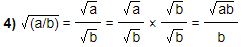• 5) Number ending in 8 can never be a perfect square.
• 6) Remember the squares and cubes of 2 to 10. This will help in easily solving the problems.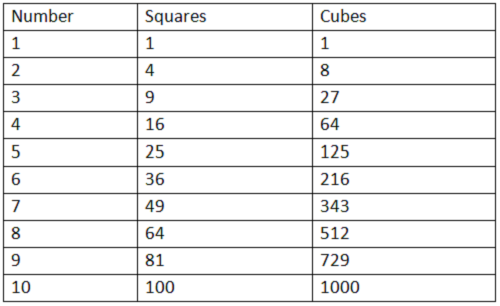### Find the numbers which have same digit in unit’s place:### Quick Tips and Tricks

1) Finding square root of 5, 4 and 3 digit numbers
How to find the square root of 5 digit number ?• Step 1: Ignore last two digits 24 and just consider first three digits.
• Step 2: Find a number near or less than 174.169 is a number near to 174 which is perfect square of 13. Hence, the number in ten’s place is 13.
• Step 3: Find the number in units’s place; 4 is the number in unit’s place. Hence, remember the table, 2 and 8 numbers have unit’s digit 4.
• Step 4: now we have to find the correct number among 2 and 8.
• Step 5 : Multiply 13 (First number) with next higher number (14) i.e 13 × 14 = 182. Number 182 is greater than the first two digits, hence consider the smallest number among 2 and 8 i.e 2. Therefore, second number is 2.
Square root of 17424 = 132
•### How to find the square root of 4 digit number?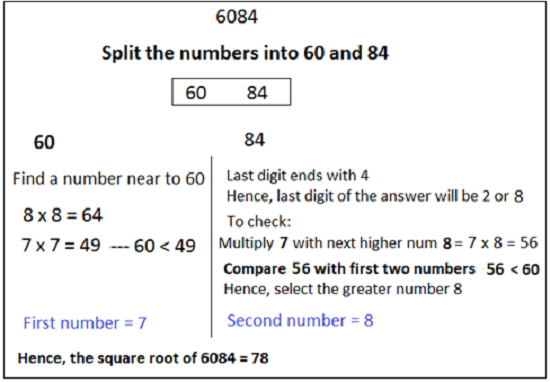• Step 1: Ignore last two digits 24 and just consider first three digits.
• Step 2: Find a number near or less than 60.49 is a number near to 60 which is perfect square of 7. Hence, the number in ten’s place is 7.
• Step 3: Find the number in unit’s place: 4 is the number in unit’s place. Hence, remember the table, 2 and 8 numbers have unit’s digit 4.
• Step 4: Now we have to find the correct number among 2 and 8.
• Step 5: Multiply 7 with next higher number (7+1) = 8 i .e 7×8 = 56. Number 56 is less than the first two digits, hence consider the largest number among 2 and 8 i.e 8. Therefore, second number is 8.
Square root of 6084 = 78

### How to find the square root of 3 digit number?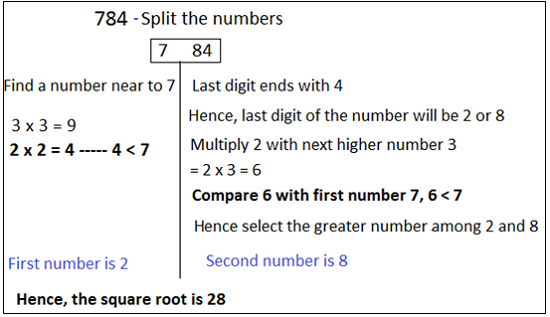• Step 1: Ignore last two digits 24 and just consider first three digits.
• Step 2: Find a number near or less than 7.4 is a number near to 7 which is perfect square of 2. Hence, the number in ten’s place is 2.
• Step 3: Find the number in unit’s place: 4 is the number in unit’s place. Hence, remember the table, 2 and 8 numbers have unit’s digit 4.
• Step 4: Now we have to find the correct number among 2 and 8.
• Step 5: Multiply 7 with next higher number (2+1 = 8) i .e 2×3 = 6. Number 6 is less than the first digits, hence consider the largest number among 2 and 8 i.e 8. Therefore, second number is 8.
Square root of 784 = 28

### 2) Finding the square of large numbers

Example: 472 = 2209
Square of 47 can be easily determined by following the steps shown below:
Step 1: Split the number 47 as 4 and 7.
Step 2: Use the formula: (a + b)2 = a2 + 2ab + b2
Here, (4 + 7)2 = 42 + 2 × 4 × 7 + 72
Without considering the plus sign, write the numbers as shown below: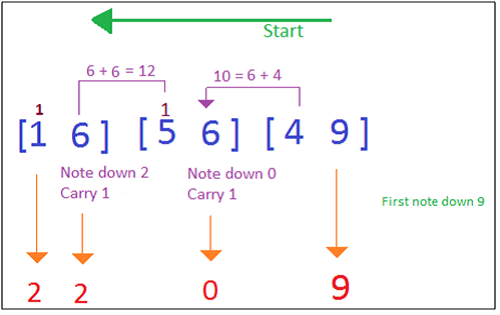• Step 1: Write down 9 from 49 and carry 4 to 56. [-----9]
• Step 2: After adding 4 to 6, we get 10. Therefore, write down zero and carry 1 (5 + 1 = 6) to 16. [----09]
• Step 3: 6 + 6 = 12, write down 2 and carry one. [---209]
• Step 4: Finally write the answer along with (1 + 1 = 2). 

### 3) Finding the cube root of 6 digit number?

Note: Cube roots of 6, 5, 4 or 3 digit numbers can be easily found out by using the same trick as used to find the square root of larger digits.
Example: 3√132651
Remember: The last 3 numbers are to cut off and the nearby cube of first remaining numbers is to be found out.

• Step 1: Split the number 132 and 651
• Step 2: 125 is the cube of 5, which is the closest number to 132. Hence, first number i.e. the number in ten’s place is 5.
• Step 3: 1 is the digit in unit’s place. Hence, the digit in unit’s place is 1.
Hence, the cube root of 132651 is 51.
• ### 4) How to find a number to be added or subtracted to make a number a perfect square ?

For easy understanding, let’s take an example. Example: 8888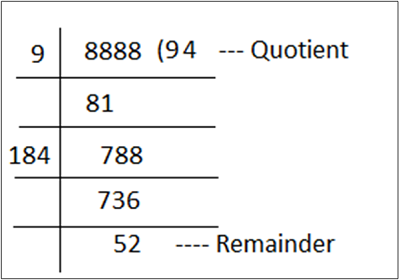• Step 1: Divide 8888 by 9. We get remainder 7.
• Step 2: Add Divisor and Quotient [9 + 9 = 18]
• Step 3: Now the next divisor will be (18 and number x) which will divide the next dividend. In this case, 4 is the number x and now the divisor becomes 184 × 4 =736.
• Step 1: This step is to be followed depend the number of digits in the dividend.

Case 1: If we have to find a number to be added to make a number perfect square, then Consider a number greater than the quotient. Her quotient is 94, hence consider 95.
942 < 8888 < 952
8836 < 8888 < 9025
Number to be added = Greater number – Given number
Number to be added = 9025 – 8888 = 137

Case 2: If we have to find a number to be subtracted to make a number perfect square, then
942 < 8888 < 952
8836 < 8888 < 9025
Number to be subtracted = Given number - Smaller number
Number to be added = 8888 – 8836 = 52

### More Educational and Fun StuffMore In-depth knowledge about what you need. Detailed about test preparation, English Writing, TOEFL, and IELTS.